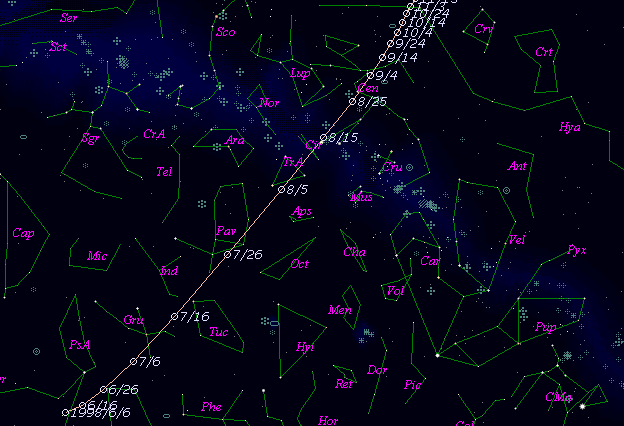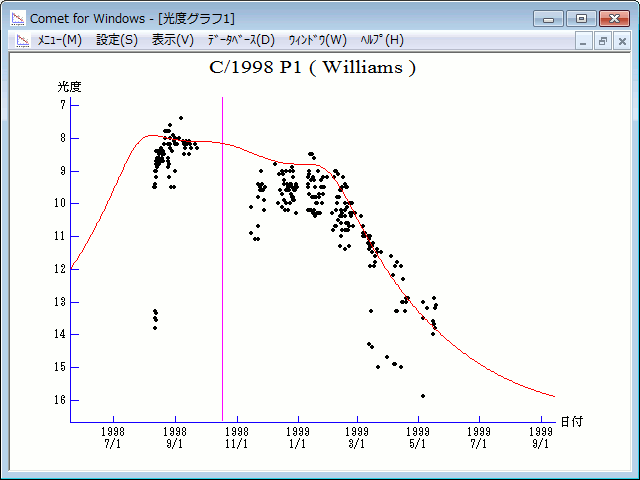# \$B%&%#%j%"%`%:WB@1(B

C/1998 P1 ( Williams )###\$B%W%m%U%#!<%k(B

 \$BH/8+F|(B 1998\$BG/(B8\$B7n(B10\$BF|(B \$BH/8+8wEY(B 9.5\$BEy(B \$BH/8+ Peter Williams (Heathcote, Australia)

###\$B###\$B50F;MWAG(B

```   The following improved orbital elements by Kenji Muraoka, are
from 398 observations  1998 Aug. 11  to  1999 May 15, including
9 Planet and 5 minor planets perturbations and non-gravitational
effect of style II ( A1 , A2 , A3 ).
The mean residual is  +/- 0.76 arc seconds.

Epoch  =  1998 Nov.  3.0  TT       JDT = 2451120.5
T  =  1998 Oct. 17.84580       +/- 0.00027 (m.e.) TT
Peri. =  294.46742                +/- 0.00040
Node  =  156.37090                +/- 0.00018   (2000.0)
Incl. =  145.72753                +/- 0.00006
q  =    1.1462029              +/- 0.0000068 AU
e  =    0.9987446              +/- 0.0000104
1/a  =   +0.0010953              +/- 0.0000091 1/AU
A1  =  +31.441                  +/- 0.283
A2  =   +2.14839                +/- 0.10776
A3  =   -1.03488                +/- 0.03907
( P  =  27588 years )
```

###\$B@1?^(B###\$B8wEYJQ2=(B

```        m1 = 6.0 + 5 log\$B&\$(B + 10.0 log r
```##### \$B50F;MWAG\$OB<2,7r<#;a\$N7W;;\$K\$h\$k\$b\$N\$G\$9!#(B \$B@1?^\$O(B StellaNavigator Ver.2.0 for Windows (\$B%"%9%H%m%"!<%D(B \$BJTCx(B / \$B%"%9%-!<=PHG6I4)(B) \$B\$G:n@.\$7\$?\$b\$N\$G\$9!#(B \$B8wEY%0%i%U\$O(BComet for Windows\$B\$G:n@.\$7\$?\$b\$N\$G\$9!#(B